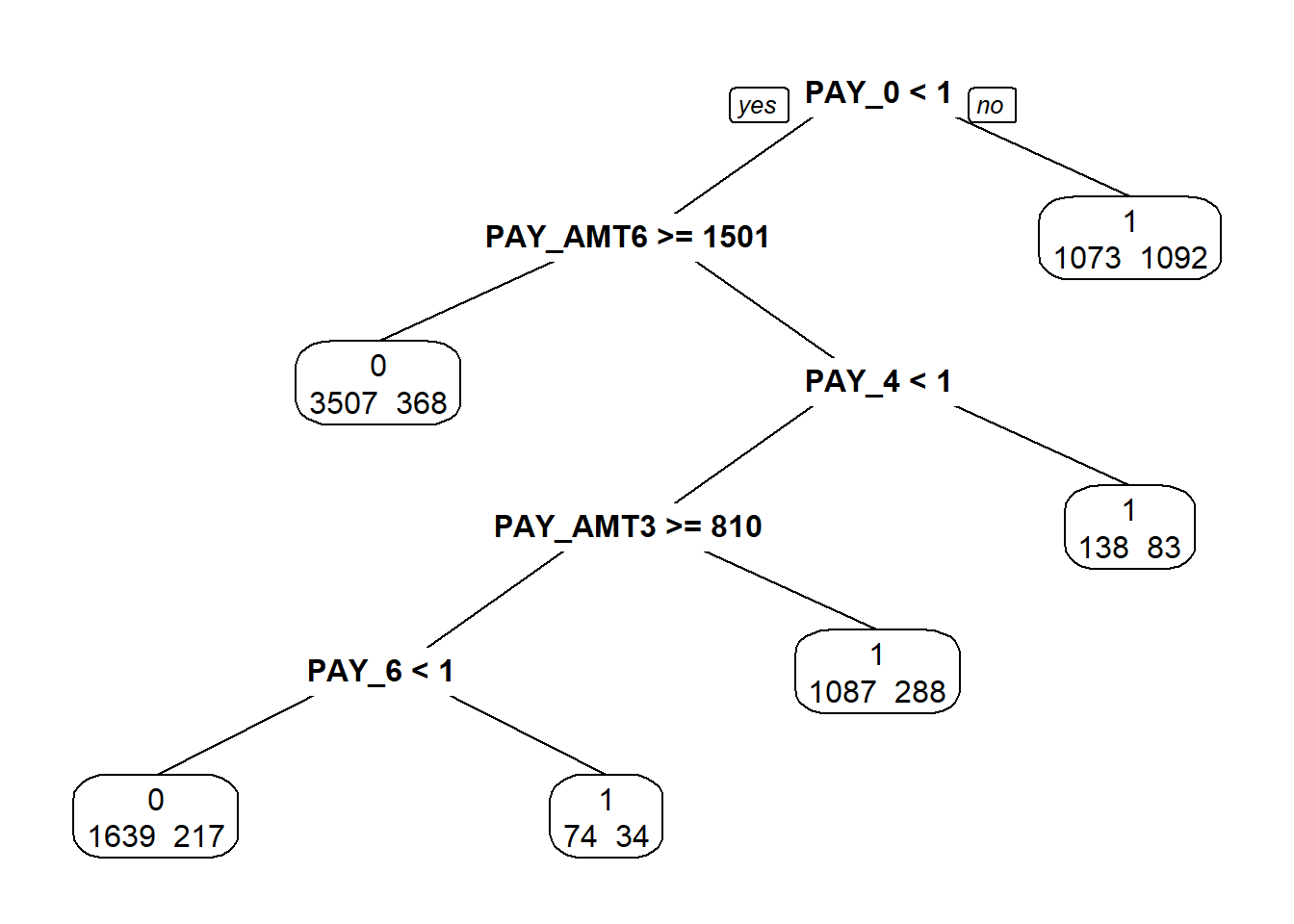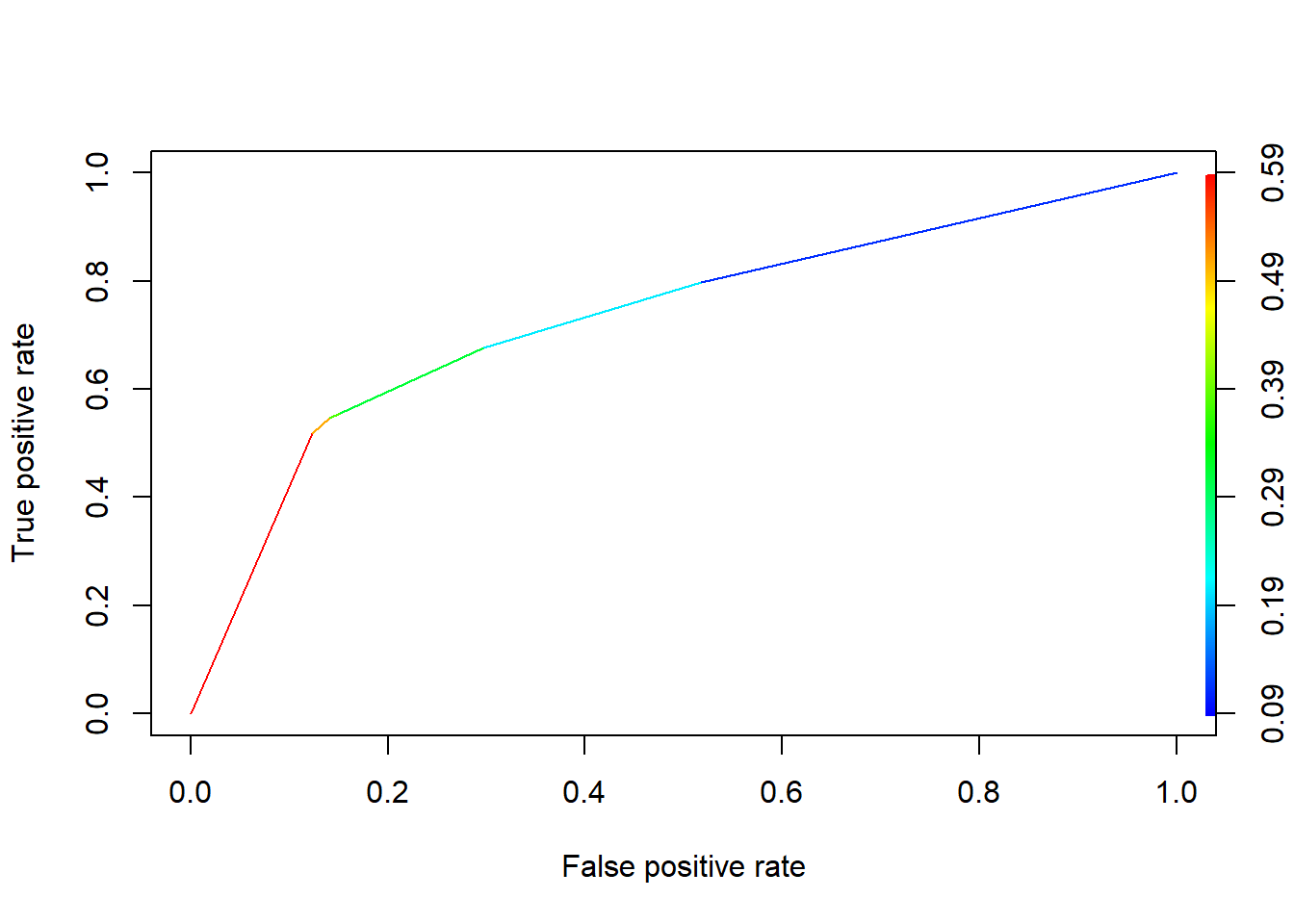In this lab we will go through the model building, validation, and interpretation of tree models. The focus will be on rpart package.

# 1 CART: classification and regression tree

CART stands for classification and regression trees:

• Regression tree: response variable Y is numerical
• Classification tree: response variable Y is categorical

For the regression tree example, we will use the Boston Housing data. Recall the response variable is the housing price. For the classification tree example, we will use the credit scoring data. The response variable is whether the loan went to default.

Note that unlike logistic regression, the response variable does not have to be binary in case of classification tree. We can use classification tree on classification problems with more than 2 outcomes.

go to top

## 1.1 Classification Trees (Credit Card Default data)

The classification trees is slightly more complicated to specify. What makes it more complicated is that we often have asymmetric cost function. In the credit scoring case it means that false negatives (predicting 0 when truth is 1, or giving out loans that end up in default) will cost more than false positives (predicting 1 when truth is 0, rejecting loans that you should not reject).

Here we make the assumption that false negative cost 5 times of false positive. In real life the cost structure should be carefully researched.

library(rpart)
library(rpart.plot)
credit_data <- read.csv(file = "https://xiaoruizhu.github.io/Data-Mining-R/lecture/data/credit_default.csv", header=T)

# rename
library(dplyr)
##
## Attaching package: 'dplyr'
## The following objects are masked from 'package:stats':
##
##     filter, lag
## The following objects are masked from 'package:base':
##
##     intersect, setdiff, setequal, union
credit_data<- rename(credit_data, default=default.payment.next.month)
# convert categorical data to factor
credit_data$SEX<- as.factor(credit_data$SEX)
credit_data$EDUCATION<- as.factor(credit_data$EDUCATION)
credit_data$MARRIAGE<- as.factor(credit_data$MARRIAGE)

index <- sample(nrow(credit_data),nrow(credit_data)*0.80)
credit_train = credit_data[index,]
credit_test = credit_data[-index,]

credit_rpart0 <- rpart(formula = default ~ ., data = credit_train, method = "class")

credit_rpart <- rpart(formula = default ~ . , data = credit_train, method = "class", parms = list(loss=matrix(c(0,5,1,0), nrow = 2)))

Note the following important differences from the regression trees:

• The method = “class” is required if the response is not declared as factors.

• The parms argument, which is a list. The most import element is the loss matrix. The diagonal elements are 0, and off-diagonal elements tells you the loss(cost) of classifying something wrong. For binary classification, the numbers in c() specify the cost in this sequence: c(0, False Negative, False Positive, 0). If you have symmetric cost, you can ignore the parms argument.

However, this tree with default cost minimizes the symmetric cost, which is misclassification rate. We can take a look at the confusion matrix.

pred0 <- predict(credit_rpart0, type="class")
table(credit_train$default, pred0, dnn = c("True", "Pred")) ## Pred ## True 0 1 ## 0 7188 330 ## 1 1385 697 Note that in the predict() function, we need type="class" in order to get binary prediction. Look at the confusion matrix, is it what we expected? Think about why the confusion matrix is like this? Therefore, for most applications (very unbalanced data), we often have asymmetric cost. Recall the example in logistic regression. In the credit scoring case it means that false negatives (predicting 0 when truth is 1, or giving out loans that end up in default) will cost more than false positives (predicting 1 when truth is 0, rejecting loans that you should not reject). Here we make the assumption that false negative cost 5 times of false positive. In real life the cost structure should be carefully researched. credit_rpart <- rpart(formula = default ~ . , data = credit_train, method = "class", parms = list(loss=matrix(c(0,5,1,0), nrow = 2))) For more advanced controls, you should carefully read the help document for the rpart function. ### 1.1.1 Printing and ploting the tree credit_rpart ## n= 9600 ## ## node), split, n, loss, yval, (yprob) ## * denotes terminal node ## ## 1) root 9600 7518 1 (0.78312500 0.21687500) ## 2) PAY_0< 0.5 7435 4950 0 (0.86684600 0.13315400) ## 4) PAY_AMT6>=1501 3875 1840 0 (0.90503226 0.09496774) * ## 5) PAY_AMT6< 1501 3560 2938 1 (0.82528090 0.17471910) ## 10) PAY_4< 1 3339 2695 0 (0.83857442 0.16142558) ## 20) PAY_AMT3>=810 1964 1255 0 (0.87219959 0.12780041) ## 40) PAY_6< 1 1856 1085 0 (0.88308190 0.11691810) * ## 41) PAY_6>=1 108 74 1 (0.68518519 0.31481481) * ## 21) PAY_AMT3< 810 1375 1087 1 (0.79054545 0.20945455) * ## 11) PAY_4>=1 221 138 1 (0.62443439 0.37556561) * ## 3) PAY_0>=0.5 2165 1073 1 (0.49561201 0.50438799) * prp(credit_rpart, extra = 1)### 1.1.2 Prediction using classification trees For a binary classification problem, as you learned in logistic regression there are 2 types of predictions. One is the predicted class of response (0 or 1), and the second type is the probability of response being 1. We use an additional argument type=“class” or type=“prob” to get these: In-sample prediction credit_train.pred.tree1<- predict(credit_rpart, credit_train, type="class") table(credit_train$default, credit_train.pred.tree1, dnn=c("Truth","Predicted"))
##      Predicted
## Truth    0    1
##     0 5146 2372
##     1  585 1497

Exercise: Out-of-sample prediction

#Predicted Class
credit_test.pred.tree1<-
table()

Usually if you want a hassle-free model, using type=“class” is enough given that you specified the loss matrix correctly in rpart.

We can get the expected loss for this tree model by defining a cost function that has the correct weights:

cost <- function(r, phat){
weight1 <- 5
weight0 <- 1
pcut <- weight0/(weight1+weight0)
c1 <- (r==1)&(phat<pcut) #logical vector - true if actual 1 but predict 0
c0 <-(r==0)&(phat>pcut) #logical vector - true if actual 0 but predict 1
return(mean(weight1*c1+weight0*c0))
}

cost(credit_train$default, predict(credit_rpart, credit_train, type="prob")) ##  0.6674479 Exercise: Out-of-sample prediction #Predicted Class credit_test.pred.tree1<- table() Exercise: Try type="prob" in prediction, what can you say about these predicted probabilities? ### 1.1.3 Calculate the actual cost using a self-defined cost function. Calculate the cost for testing sample using above cost function cost(credit_test$default,  predict(credit_rpart, credit_test, type="prob"))
##  0.6852083

### 1.1.4 Comparing this classification tree with logistic regression

We can compare this model’s out-of-sample performance with the logistic regression model with all variables in it.

#Fit logistic regression model
credit_glm <- glm(default~.,
data = credit_train,
family=binomial)
#Get binary prediction
credit_test_pred_glm <- predict(credit_glm, credit_test, type="response")
#Calculate cost using test set
cost(credit_test$default, credit_test_pred_glm) ##  0.6858333 #Confusion matrix table(credit_test$default, as.numeric(credit_test_pred_glm>1/6), dnn=c("Truth","Predicted"))
##      Predicted
## Truth   0   1
##     0 884 966
##     1 136 414

Exercise: Comparison for in-sample performance. Which model do you think is better?

go to top

### 1.1.5 ROC Curve and Cut-off Probability for Classification trees

Recall that ROC Curve gives you the trade-off between hit rate (1 - false positive) and false negative, and area under the curve (AUC) can be used as a measure of how good the binary classification model performs when you do not know the cost function.

To get ROC curve, we get the predicted probability of Y being 1 from the fitted tree. The additional cp parameter controls the complexity of tree. Here we change it from its default 0.01 to a smaller value to grow a more complex tree than just the root node (if you use the default the tree you get will tell you to classify everything as 0). More discussion on this in the next section.

credit_rpart <- rpart(formula = default ~ .,
data = credit_train,
method = "class",
parms = list(loss=matrix(c(0,5,1,0), nrow = 2)))
#Probability of getting 1
credit_test_prob_rpart = predict(credit_rpart, credit_test, type="prob")

credit_test_prob_rpart has 2 columns, the first one is prob(Y) = 0 and the second prob(Y) = 1. We only need the second column because they add to 1 for binary classification.

To get ROC curve we use

install.packages('ROCR')
library(ROCR)
pred = prediction(credit_test_prob_rpart[,2], credit_test$default) perf = performance(pred, "tpr", "fpr") plot(perf, colorize=TRUE)Area under the curve is given by (do not worry about the syntax here): slot(performance(pred, "auc"), "y.values")[] ##  0.7334393 For a given cut-off probability, the 0/1 prediction result can be calculated similar to what you do in logistic regression credit_test_pred_rpart = as.numeric(credit_test_prob_rpart[,2] > 1/(5+1)) table(credit_test$default, credit_test_pred_rpart, dnn=c("Truth","Predicted"))
##      Predicted
## Truth    0    1
##     0 1301  549
##     1  178  372

You can refer to the last section on specifying a loss matrix, rpart will automatically generate decision rules with your cost structure taken into consideration.

Exercise: Draw the ROC curve for training sample.

## 1.2 Things to remember

• Use rpart() to fit regression and classification trees.

• Know how to interpret a tree.

• Use predict() for prediction, and how to assess the performance.

• Know how to use Cp plot/table to prune a large tree.

go to top